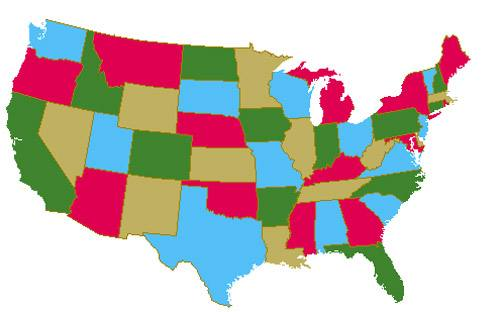# How Many Colors For The Map

By Math Is Fun, August 14, 2022

Son was filling colors in his drawings, which reminded me of some fun in math: “Do you know how many colors are needed for a world map?” I asked son.

“Many colors?”

“Let me ask the question better. There are many countries in the world. In the map, we want to use different colors for any two countries that share a border, so that you can easily recognize different countries. What’s the smallest number of colors you need?”

“If a country has 10 neighboring countries, then we need 11 colors right?”

“No. For example, the United States has Canada and Mexico as neighbors. But Canada and Mexico do not share a border. They can be the same color.”

Son now understood the question. I printed a blank Africa map as an example for him to fill in the colors.

“It seems 3 colors are enough.” Son said after trying for a while.

“It turns out 3 colors are not enough.” I drew an example.

In this example, each of the four countries is bordering another country, so we need four colors. So are 4 colors enough? This was actually one of the most famous conjectures in the history of math. A conjecture is a seemingly-true statement that is not mathematically proved. In plain words, a conjecture is an unsolved math problem.

The history of the four-color conjecture was an interesting story by itself. It was first proposed in 1852 by Francis Guthrie, a then-English college math student. He later migrated to South Africa and became a mathematician. He proposed this problem when coloring an England map.

The four-color conjecture is a problem of Topology, a math sub-branch. We mentioned Topology before in our Mobius band article.

The four-color problem seemed pretty straightforward. And many mathematicians immediately tried to solve it after it was proposed, thinking it shouldn’t be too difficult. It turned out to be not easy. Finally, in 1879, Alfred Kempe provided a proof and people thought that was it. However, 11 years later, it was found incorrect.

It was not until 1976, 124 years after the four-color conjecture was first proposed, did two professors from UIUC prove the conjecture, with the help of computers. The four-color conjecture became the four-color theorem. A math theorem is a statement that has been proved. This was the first time a major mathematical theorem was proved with the assistance of computers.

To celebrate this major achievement, the math department of UIUC used this postage stamp for several years for outgoing mails. It said “Four Colors Suffice”!